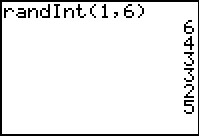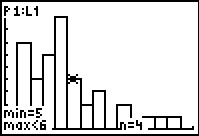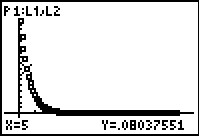# Activities

•##### Download

•• ##### Subject Area

• Math: Statistics: Normal Distributions
• Math: Statistics: Probability and Random Variables

• ##### Author9-12

45 Minutes

• ##### Device
• TI-83 Plus Family
• TI-84 Plus
• TI-84 Plus Silver Edition
•TI-84 Plus C Silver Edition
•TI-84 Plus CE
• ##### Report an Issue

Geometric Distributions#### Activity Overview

Students simulate a geometric distribution of rolling a die to determine experimental probabilities and calculate theoretical probabilities.

#### Key Steps

•Problem 1 introduces students to the geometric distribution. They to determine whether several given situations represent a geometric distribution.

In the second problem, students will investigate the probability of the first 5 appearing on the fourth roll. Students will use the Random Integer command to simulate the geometric distribution.

•They will combine their results with other classmates and calculate the experimental probability. Students will use a histogram to help calculate probabilities and form conclusions.

•In Problem 3, students will explore the theoretical probabilities of a geometric distribution using the Geometric Pdf command. They will then derive the formula for calculating the probability of the first 5 appearing on the nth roll. Then students will graph the probabilities as a scatter plot and determine the regression equation. They will algebraically verify that the formula and the regression equation are the same.